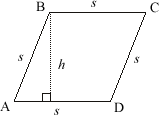index: click on a letter A B C D E F G H I J K L M N O P Q R S T U V W X Y Z A to Z index index: subject areas numbers & symbols sets, logic, proofs geometry algebra trigonometry advanced algebra & pre-calculus calculus advanced topics probability & statistics real world applications multimedia entrieswww.mathwords.com about mathwords website feedback

Area of a Rhombus

Formulas are given below. Note that the diagonals of a rhombus are perpendicular (as is the case with all kites).

 Rhombus s = side length of rhombus h = height of rhombus d1 = long diagonal of rhombus d2 = short diagonal of rhombus Area = hs          = s2 sin A          = s2 sin B          = (½) d1d2